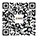܄

# 预测性客户分析之客户终生价值（Part 3）

【数据猿导读】 本篇将会介绍如何用线性回归模型，基于老客户历史数据与客户生命周期的关联关系，建立线性回归模型，从而预测新客户的终生价值，进而开展针对性的活动“我们80%的收入来自于20%的客户。卖给现有客户的成本比寻找一个新的客户低10倍。”

• 过去的收入如何预示未来的收入？

• 我们过去最好的客户在未来还会是我们最好的客户吗？

• 这是我们手中的结果吗？from pandas import Series, DataFrame
import pandas as pd
import numpy as np
import os
import matplotlib.pylab as plt
from sklearn.model_selection import train_test_split
from sklearn.linear_model import LinearRegression
import sklearn.metrics
raw_data = pd.read_csv(“history.csv”)
raw_data.dtypes

``````CUST_ID int64
MONTH_1 int64
MONTH_2 int64
MONTH_3 int64
MONTH_4 int64
MONTH_5 int64
MONTH_6 int64
CLV int64
dtype: object``````

raw_data.head()cleaned_data = raw_data.drop(“CUST_ID”,axis=1)

cleaned_data .corr()[‘CLV’]predictors = cleaned_data.drop(“CLV”,axis=1)
targets = cleaned_data.CLV
pred_train, pred_test, tar_train, tar_test = train_test_split(predictors, targets, test_size=.1)
print( “Predictor — Training : “, pred_train.shape, “Predictor — Testing : “, pred_test.shape)

``Predictor - Training : (90, 6) Predictor - Testing : (10, 6)``

#Build model on training data

model = LinearRegression()

model.fit(pred_train,tar_train)

print(“Coefficients: \n”, model.coef_)

print(“Intercept:”, model.intercept_)

#Test on testing data

predictions = model.predict(pred_test)

predictions

sklearn.metrics.r2_score(tar_test, predictions)

``````Coefficients:
[ 34.59195048  11.53796271  15.17878598  11.72051702   8.60555913
5.44443443]
Intercept: -199.535985333```
```

Out:

``0.91592106093124581``

new_data = np.array([100,0,50,0,0,0]).reshape(1, -1)
new_pred=model.predict(new_data)
print(“The CLV for the new customer is : \$”,new_pred)

``The CLV for the new customer is : \$ 4018.59836236``

[2017/12/19]

More>

[2017/12/18-22]

More>

[2017/12/18-22]

More>

[2017/12/18-22]

More>

[2017/12/13-20]

More>

[2017/12/12-19]

More>

## 人物专访more >## 大数据学堂more >## 大家都在搜